# Digital Line Coding

## Line Coding

Digital Line Coding is a special coding system used for the transmission of digital signal over a transmission line.

It is the process of converting binary data to a digital signal.

The data, text,numbers, graphical images, audio and video which are stored in computer memory are in the form of binary data or sequence of bits. Line coding converts these sequences into digital signal.

### Properties of Line Coding

1. Transmission bandwidth : For a line-code, the transmission bandwidth must be as small as possible.
2. Power efficiency : For a given bandwidth and a specified detection error probability, the transmitted power for a line-code should be as small as possible.
3. Probability of Error : The probability of error is much reduced.
4. Error detection and correction capability : It must be possible to detect and preferably correct detection errors.  For example, in a bipolar case, a signal error will cause bipolar violation and thus can easily be detected.
5. Power density : It is desirable to have zero power spectral density (PSD) at ω =0 (i.e. , d.c) since ac coupling and transformers are used at the repeaters. Significant power in low-frequency components causes dc wander in the pulse stream when ac coupling is used.The a.c . coupling is required since the dc paths provided by the cable pairs between the repeater sites are used to transmit the power required to operate the repeaters.
6. Adequate timing content : It must be possible to extract timing or clock information from the signal.
7. Transparency : It must be possible to transmit a digital signal correctly regardless the pattern of 1’s and 0’s.

### Types of Line Coding

There are 3 types of Line Coding

• Unipolar
• Polar
• Bi-polar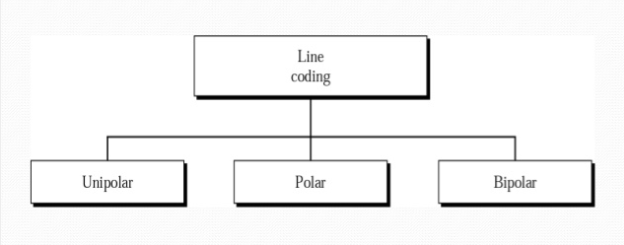Fig.1 : Types of Line Coding

### Unipolar Signaling

The Unipolar signaling is also known as On-Off Keying or simply OOK.

Here, a ‘1’ is transmitted by a pulse and a ‘0’ is transmitted by no pulse. i.e.,the presence of pulse represents a ‘1′ and the absence of pulse represents a ‘0′.

There are two common variations in Unipolar signaling :

In this type of unipolar signaling, a High (1)in data is represented by a positive pulse called as Mark, which has a duration T0 equal to the symbol bit duration. A Low(0) in data input has no pulse.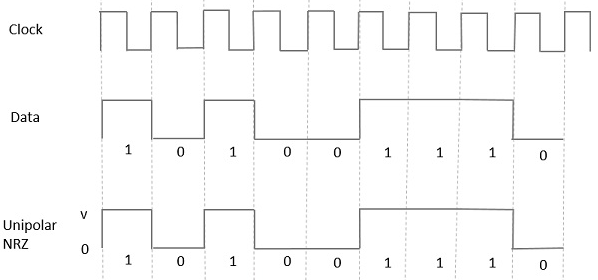The advantages of Unipolar NRZ are −

• It is simple.
• A lesser bandwidth is required.

The disadvantages of Unipolar NRZ are −

• No error correction capability.
• Presence of low frequency components may cause the signal droop.
• No clock is present for the ease of synchronization.
• It is not transparent.Loss of synchronization is likely to occur especially for long strings of 1s and 0s.

In this type of unipolar signaling, a High in data, though represented by a Mark pulse, its duration T0 is less than the symbol bit duration. Half of the bit duration remains high but it immediately returns to zero and shows the absence of pulse during the remaining half of the bit duration.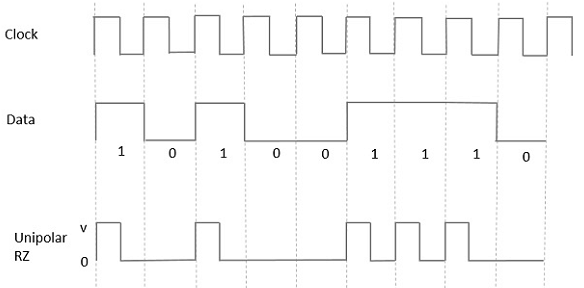• simple to implement.
• The spectral line present at the symbol rate can be used as a clock.

• No error correction capability.
• Occupies twice the bandwidth as unipolar NRZ.
• The signal droop is caused at the places where signal is non-zero at 0 Hz.
• Not transparent.

### Polar Signaling

There are two methods of Polar Signaling. Such as:

• Polar NRZ
• Polar RZ

### Polar NRZ

In this type of Polar signaling, a High (1)in data is represented by a positive pulse, while a Low (0) in data is represented by a negative pulse.

This is shown in fig.4 below.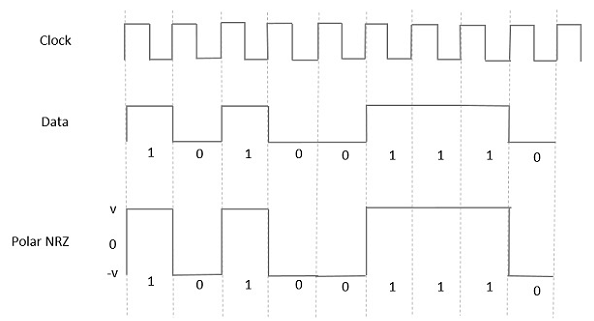Fig.4 : Polar NRZ

The advantages of Polar NRZ are −

• Simplicity in implementation.
• No low-frequency (DC) components are present.

The disadvantages of Polar NRZ are −

• No error correction capability.
• No clock is present for the ease of synchronization.
• Signal droop is caused at the places where the signal is non-zero at 0 Hz.
• It is not transparent.

### Polar RZ

In this type of Polar signaling, a High (1)  in data, though represented by a Mark pulse, its duration T0 is less than the symbol bit duration. Half of the bit duration remains high but it immediately returns to zero and shows the absence of pulse during the remaining half of the bit duration.

However, for a Low (0) input, a negative pulse represents the data, and the zero level remains same for the other half of the bit duration.

This is shown in fig.5 below .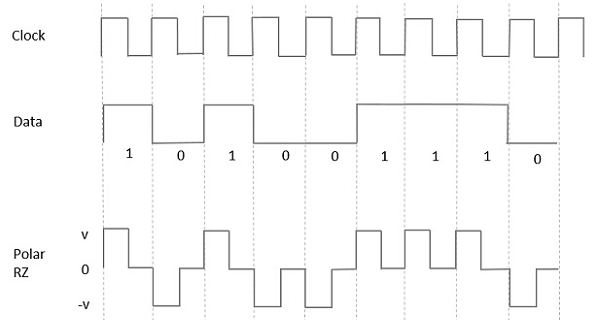Fig.5 : Polar RZ

The advantages of Polar RZ are −

• Simplicity in implementation.
• No low-frequency (DC) components are present.

The disadvantages of Polar RZ are −

• No error correction capability.
• No clock is present for the ease of synchronization.
• Occupies twice the bandwidth of Polar NRZ.
• The signal droop is caused at places where the signal is non-zero at 0 Hz.

### Bipolar Signaling

This is an encoding technique which has three voltage levels such as : +, – and 0. Such a signal is known as duo-binary signal.

An example of this type is Alternate Mark Inversion (AMI).

For a 1, the voltage level gets a transition from + to – or from – to +, having alternate 1s to be of equal polarity. A 0 will have a zero voltage level.

There are  two types in this method. such as :

• Bipolar NRZ
• Bipolar RZ

As  we have already discussed the difference between NRZ and RZ, the same way here too.

The following figure clearly describes this.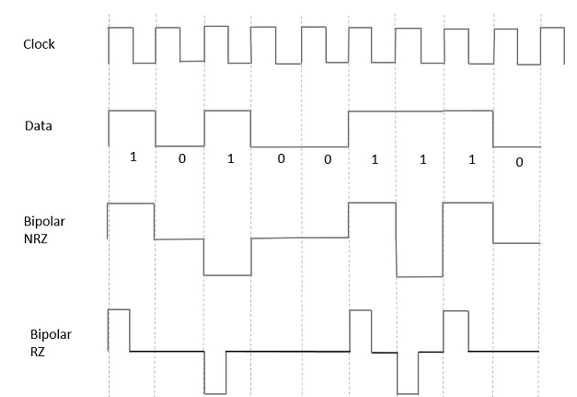Fig.6

In the above fig.6, both the Bipolar NRZ and RZ waveforms are shown.

The pulse duration and symbol bit duration are equal in NRZ type, while the pulse duration is half of the symbol bit duration in RZ type.

• Simplicity in implementation.
• No low-frequency(DC) components are present.
• Occupies low bandwidth than unipolar and polar NRZ techniques.
• Signal drooping doesn’t occur here, hence it is suitable for transmission over AC coupled lines.
• A single error detection capability is present here.

• No clock is present.
• It is not transparent.i.e.,long strings of data causes loss of synchronization.

### Power Spectral Density

The function which shows how the power of a signal got distributed at various frequencies, in the frequency domain is known as Power Spectral Density (PSD).

PSD is the Fourier Transform of Auto-Correlation . It is in the form of a rectangular pulse.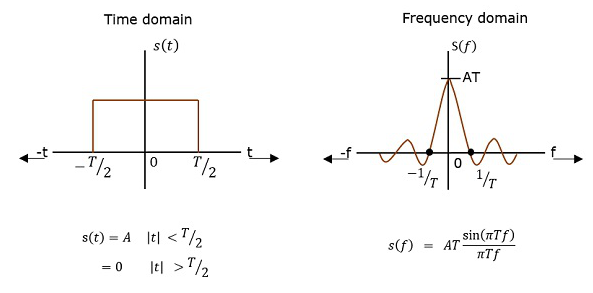Fig.7

### PSD Derivation

According to the Einstein-Wiener-Khintchine theorem, if the auto correlation function or power spectral density of a random process is known, the other can be found exactly.

Hence, to derive the power spectral density, we shall use the time auto-correlation (Rx(τ)) of a power signal x(t) as shown below.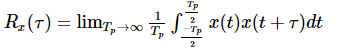Since x(t) consists of impulses, (Rx(τ))  can be written as:Where: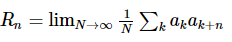Getting to know that  R= R-n , for real signals, we have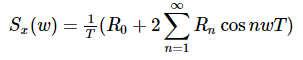Since the pulse filter has the spectrum of (ω)↔f(t), we have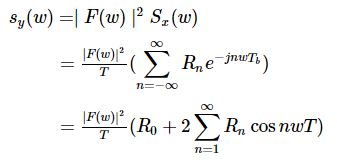Now we can find the PSD of various line codes using this equation for Power Spectral Density .